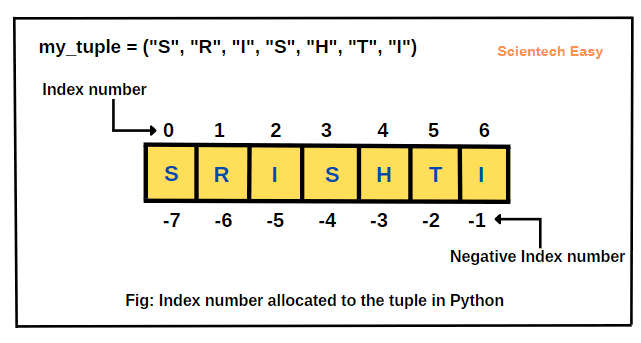# How to Access Tuple Elements in Python

In this tutorial, we will learn how to access tuple elements in Python with the help of examples. There are different ways by which we can access elements of a tuple in Python. They are as:

• Indexing
• Negative indexing
• Slicing

Let’s understand each method one by one with examples.

## Access Tuple Elements using Indexing in Python

When we create a tuple in Python, elements of a tuple automatically get allocated with an index number. The index number for each element in the tuple is unique and the number always gets started from zero.

That is, the first element is on the index zero, the second element is on index 1, and so on. With the help of indexing, we can access each element individually.

We can use the index operator or square brackets [ ] to access items or elements in a tuple. Here, the square brackets show the index of the element we want to access.

If you know how to access elements in a list through indexing, you can use the same method to access elements in a tuple. The general syntax to access elements of a tuple in Python is as follows:

```tuple_name[index]
```

In this syntax, index inside brackets should always be an integer value that indicates element or value being selected or accessed. If you access an element outside the scope of the indexed elements, it will generate an IndexError. In addition, accessing an index with a non-integer numeric value will generate a NameError.

Example 1:

Let’s take an example in which we will create a tuple having seven elements with index values from 0 to 6, as shown in the below figure.Let’s write program code to access first, second, third, and the last elements of the above tuple.

```# Python program to access elements of a tuple.
my_tuple = ("S", "R", "I", "S", "H", "T", "I")

# Accessing elements of the tuple.
print("Accessing first element: ", my_tuple)
print("Accessing second element: ", my_tuple)
print("Accessing third element: ", my_tuple)
print("Accessing last element: ", my_tuple)
```
```Output:
Accessing first element:  S
Accessing second element:  R
Accessing third element:  I
Accessing last element:  I
```

Example 2:

If we try to access an element other than the index number, or if the index number is more than the number of elements in the tuple, it will raise an error named IndexError. Look at the below example code.

```my_tuple = ("S", "R", "I", "S", "H", "T", "I")
# Accessing elements of the tuple out of range.
print(my_tuple)
```
```Output:
IndexError: tuple index out of range
```

Example 3:

If we use float or other type value as an index number, it will give an error named TypeError. Look at the below code.

```# Creating a tuple having five elements.
my_tuple = ("S", "R", "I", "S", "H")

# Accessing elements of the tuple with a float index number.
print(my_tuple[3.5])
```
```Output:
TypeError: tuple indices must be integers or slices, not float
```

## Accessing Tuple Elements using Negative Indexing

Python also allows to use negative indexing for its sequence of elements, as shown in the above figure. The index of -1 represents the last element, -2 to the second last element, and so on. It is just the reverse way of accessing elements of a tuple. Look at the example code below.

Example 4:

```# Python program to access elements of a tuple using negative index value.
my_tuple = ("S", "R", "I", "S", "H", "T", "I")

# Accessing elements of the tuple with negative index number.
print("Accessing last element: ", my_tuple[-1])

Accessing last element:  I
Accessing second last element:  T
Accessing first element:  S
```
```Output:
Accessing last element:  I
Accessing second last element:  T
Accessing first element:  S
```

In this tutorial, we have discussed how to access tuple elements in Python with the help of important examples. Hope that you will have understood the basic concept of accessing elements of tuple.